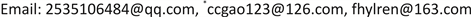1中国海洋大学数学科学学院，山东 青岛

2潍坊学院信息与控制工程学院，山东 潍坊1. 引言

{ E ( x , t ) x ˙ = f ( x , u , t ) y = g ( x , u , t ) (1)

2. 变结构控制与广义系统概述2.1. 变结构概述

2.2. 广义系统概述

3. 时滞广义变结构控制系统研究方法

3.1. 滑模近似法

{ E x ˙ ( t ) = ( A + Δ A ) x ( t ) + ( B + Δ B ) x ( t − τ ) + ( F + Δ F ) u ( t ) + D g ( t ) x ( t ) = φ ( t ) , t ∈ [ − τ , 0 ] (2)

3.2. 受限综合法

{ E x ˙ ( t ) = A ( t ) x ( t ) + f ( t , x ( t ) , x ( t − τ ) ) + B ( t ) u ( t ) x ( t ) = φ ( t ) , t ∈ [ − τ , 0 ] (3)

1) 若 r a n k ( B ( t ) , f ) = r a n k ( B ( t ) ) ，则系统(3)受限等价于：

{ x ˙ ( t ) = A 11 ( t ) x 1 ( t ) + A 12 ( t ) x 2 ( t ) 0 = A 21 ( t ) x 1 ( t ) + A 12 ( t ) x 2 ( t ) + f 2 ( t , x 1 ( t ) , x 2 ( t ) , x 1 ( t − τ ) , x 2 ( t − τ ) ) + B 2 ( t ) u ( t ) [ x 1 ( t ) x 2 ( t ) ] = P − 1 φ ( t ) = [ φ 1 ( t ) φ 2 ( t ) ] , t ∈ [ − τ , 0 ] (4)

2) 若 r a n k ( B ( t ) , f ) ≠ r a n k ( B ( t ) ) ，则系统(3)受限等价于：

(5)

3.3. 软变结构控制法

x ˙ ( t ) = A x ( t ) + b u ( t ) (6)

| u ( t ) | ≤ u 0 (7)

u ( t ) = − k p T x ( t ) , p = 1 , 2 , ⋯ , k (8)

2) 动态软变结构控制：假设所选择的策略参量 p 满足 p ˙ = f ( p , x ) ，则设计的控制器为：

u = − g T x − p h T x (9)

x ˙ = ( A − b g T ) x − p b h T x = ( A 0 − p b h T ) x (10)

3) S-型函数软变结构控制：构造两个向量 k 1 ∈ R n , k 2 ∈ R n ，使得

u = u 1 + u 2 (12)

x ˙ = ( A − b k 1 T − ρ φ b k 2 T ) x Δ _ _ A ¯ ( ρ ) x (13)

3.4. 趋近律法3.4.1. 趋近律的研究状况

s 2 ( k + 2 ) = f [ s 2 ( k + 1 ) ] f [ s 2 ( k ) ] s 2 ( k ) − α sgn [ s 2 ( k + 1 ) ] − f [ s 2 ( k + 1 ) ] α sgn [ s 2 ( k ) ] + c 2 T A b α ¯ ( k ) + c 2 T A b d ( k ) − d 2 (14)

S ˙ = − k | s | α N | s | s i g n ( S ) (15)

3.4.2. 理想趋近律与扰动补偿趋近律

3) 稳定于切换面时速度趋于零，即 lim k → ∞ s ( k + 1 ) = s ( k ) 。

s ( k + 1 ) = ( 1 − q T ) s ( k ) − ε T s g n s ( k ) + c T g ( k ) − ρ s g n s ( k ) − ∑ i = 2 k [ s ( i ) − I ( α ) s ( i − 1 ) + ρ s g n s ( i − 1 ) ] (16)

3.5. 受限等价法

1) 非结构式扰动的单时滞情况；2) 具有结构式扰动的单时滞情况；3) 非结构式扰动的多时滞情况；4) 具有结构式扰动的多时滞情况。

3.6. 自适应滑模控制法

u ( k ) = − ( C B ) − 1 [ C B K x ( k ) + K s i g n ( s ( k ) ) + s i g n ( s ( k ) ) A x ] (17)

s i g n ( s ( k ) ) = [ s i g n ( s 1 ( k ) ) ⋮ s i g n ( s m ( k ) ) ] ， K = [ K 1 0 0 0 ⋱ 0 0 0 K m ]

Gracia  等研究了一类机器人自适应滑模控制问题，通过考虑和比较几种自适应开关增益，来分析所产生的不稳定性及其其他性能；Liu  等研究的是一类离散非线性系统的无记忆自适应滑模控制，设计的控制器如下所示：

u k = u k − 1 + Φ 2 , k − 1 ( e k − Δ y k Φ 1 , k − ε ¯ k + 1 ( η θ k + 1 − η ) − ε _ k + 1 η θ k + 2 − η ϑ k + 1 ) + Φ 2 , k − 1 Λ s sign ( s k ) (18)

u = φ − 1 [ q ¨ d + α e ˙ q p + β e q 2 p − q − f + λ s + φ T A ^ s ‖ s ‖ ] (19)

Zhang  等是采用鲁棒自适应积分滑模控制方法，提出了一种基于动态滞后补偿的控制方法，研究了一类具有时间滞后和不确定性的系统的稳定性问题，设计了一种自适应律来估计积分滑模控制器的增益，从而削弱了系统抖振，且不再需要先验的不确定性上界有界的约束条件。采用自适应滑模控制法来设计控制器，不仅能够保证系统的鲁棒性，而且能够对系统的不确定性和外界干扰性进行补偿。

3.7. 随机广义控制法

4. 几个新的研究方向

1) 模糊时滞广义VSC系统的分析与综合；2) 冗余时滞广义VSC系统的分析与综合；3) 不确定时滞广义VSC系统的分析与综合；4) 随机时滞广义VSC系统的分析与综合；5) 分布参数时滞广义VSC系统的分析与综合；6) 时滞广义VSC系统的定向分析综合与应用；7) 时滞广义VSC系统的智能控制理论与应用；8) 时滞广义VSC系统在各类实际中的应用分析；9) 基于神经网络的时滞广义VSC系统的应用研究；10) 分数阶时滞广义VSC系统的分析与综合；11) 耦合时滞广义VSC系统的分析与综合；12) 混沌时滞广义VSC系统的分析与综合等。

5. 结语